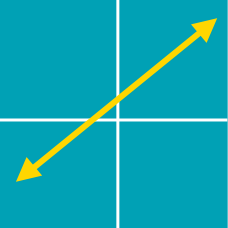Algebra

# Finding Points on a Line

The point $$(12, k)$$ lies on the line $$y = 2x + 7$$. What is the value of $$k$$?

If $$P=(a, 3)$$ is a point on the line $x+y-11=0,$ what is the value of $$a$$?

If the graph of $$y=7x-20$$ passes through two points $$A=(-1,p)$$ and $$B=(q,14),$$ what is the value of $$p+28q?$$

How many points are there on the line $$x+2y=9$$ such that the $$x$$- and $$y$$-coordinates are both non-negative integers?

If $$P=(5, a)$$ is a point on the line $3x+y-22=0,$ what is the value of $$a$$?

×